## Linear Methods of Applied Mathematics Evans M. Harrell II and James V. Herod

(Some remarks for the instructor).

version of 11 May 2000

## The fundamental solution of the heat equation

In this chapter we return to the subject of the heat equation, first encountered in Chapter VIII. The heat equation reads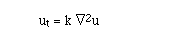(20.1)
and was first derived by
Fourier (see derivation). The same equation describes the diffusion of a dye or other substance in a still fluid, and at a microscopic level it results from random processes. We shall use this physical insight to make a guess at the fundamental solution for the heat equation.

The term fundamental solution is the equivalent of the Green function for a parabolic PDE like the heat equation (20.1). Since the equation is homogeneous, the solution operator will not be an integral involving a forcing function. Rather, the solution responds to the initial and boundary conditions.

In order to limit the complications, we shall begin with the problem of diffusion in one space dimension, and place the boundaries at infinity, so the conditions will not be looked at explicitly. (Implicitly, there is a condition of boundedness; if we allowed the addition of uunbounded(t,x) = x, for example, the problem would be in the second Fredholm alternative.)

XX.1. A well-posed problem for the one-dimensional heat equation would be of the form:

for -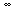< x <and 0 < t <, with given initial data:

u(0,x) = f(x). The solution depends linearly on the initial data f(x), since (20.1) is a homogeneous linear equation, so we can hope that the solution operator is an integral operator of the form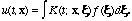(20.3)

Definition XX.2. Suppose that A is a linear operator on a suitable space of functions of x, and that u satisfies

ut = A u, u(0,x) = f(x).                                         (20.4) If there is a solution operator of the form (20.3), then K(t; x,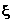) is referred to as a fundamental solution of (20.4).

Au usual for Green functions, there is an intuitive physical meaning to the fundamental solution: It is the solution with initial data

u(0,x) =(x-). Once we have this solution, any solution with other initial data can be thought of as a continuous superposition of solutions with these simpler, but singular, initial conditions.

There are several ways to derive the fundamental solution of the heat equation in unrestricted space. For example, we could apply either the Fourier or Laplace transforms, in the spatial variables x, to obtain a differential equation involving only t, and then transform back. Instead, here we use the physics of diffusion as a hint to form an ansatz for the solution and then make a calculation.

We take the opportunity at this stage to scale time to make the constant in the heat equation k=1; the mathematically natural time variable is t' = kt.)

The hint is that the underlying mechanism is some sort of random process which is isotropic, unrestricted in space, and invariant in time, then we might expect that the density u(t,x) could be a Gaussian distribution, and once it has this distribution, it should remain a Gaussian, although it will spread out as time goes by.

A Gaussian density on the x-axis, centered at x=0, is of the form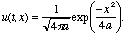(20.5) The normalization is taken so that the integral of the Gaussian density is 1. If we are right, we should be able to find a function a(t) so that the function (20.5) solves the one-dimensional heat equation. Let's plug in and see what we get: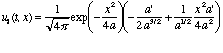whereas a slightly longer calculation reveals that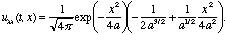These differ only by the factor a'(t). Gratifyingly, the ansatz works with the very simple choice a(t) = t. We now take a closer look at the fundamental solution we have found: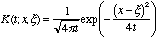is known as the one-dimensional heat kernel.

(See the exercises for some alternative ways to derive the heat kernel.)

We have not yet explained in what sense the heat kernel approaches the delta function as t tends to 0. Notice that the Gaussian distribution of the heat kernel becomes very narrow when t is small, while the height scales so that the integral of the distribution remains one. Hence if we integrate it by any continuous, bounded function f(pix/bfxi.gif) and take the limit, we will in fact get f(x).

In the contrary limit, the distribution becomes very broad, corresponding to our experience that a diffusing substance tends to a widespread, nearly uniform, density.

Model Problem XX.4. Suppose k=1 in (20.2) and the initial condition is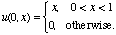Solve for u(t,x).

Solution. We perform the integral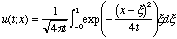with software, and find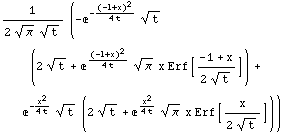Here Erf denotes the error function of statistics,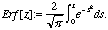An additional convenient feature of the heat kernel is that the extension to many dimensions is immediate, as can be verified by directly plugging into the heat equation:

Definition XX.5. The function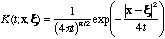is the heat kernel for Rn.

In addition to helping us solve problems like Model Problem XX.4, the solution of the heat equation with the heat kernel reveals many things about what the solutions can be like. For example, if f(x) is any bounded function, even one with awful discontinuities, we can differentiate the expression in (20.3) under the integral sign. In fact, we can differentiate as often as we like. This means that an initially irregular distribution of temperature or a diffusing substance, is instantaneously smoothed out. Perhaps this is not too surprising when we think that the heat kernel itself solves the heat equation, and changes instantaneously from a delta function to a smooth, Gaussian distribution.

Model Problem XX.6. Again consider the one-dimensionalheat equation with k=1, but include a source of heat which is constant in time:

ut - uxx = f(x),                                         (20.6)
u(0,x) = g(x)
for two given functions f(x) and g(x) defined for -< x <Solution. Let's try to reduce this problem to the familiar (20.1) solved by the fundamental solution we already know, by subtracting something off to make (20.6) homogeneous.

One way to do this is to write w(t,x) = u(t,x) + F(x), where F''(x) = f(x). That is, F(x) is any second integral of f(x). By substituting, we find that

wt - wxx = ut - uxx - f(x) = 0,
w(0,x) = g(x) + F(x).
Hence the solution is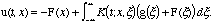Exercises XX
1. Solve the initial-value problem for -< x <: ut = uxx,
u(0,x) = exp(-|x|)
2. Use the free heat kernel and the method of images of Chapter XIX to find the fundamental solution for the initial-boundary-value problem for 0 < x <: ut = uxx,
u(t,0) = 0
u(0,x) = g(x) (a given function)
3. Use the free heat kernel and the method of images of Chapter XIX to find the fundamental solution for the initial-boundary-value problem for 0 < x <: ut = uxx,
ux(t,0) = 0
u(0,x) = g(x) (a given function)
4. Use the free heat kernel and the method of images of Chapter XIX to find the fundamental solution for the initial-boundary-value problem for 0 < x < 1: ut = uxx,
u(t,0) = u(t,1) = 0
u(0,x) = g(x) (a given function)
Compare the infinite-series you obtain with the solution using separation fo variables from Chapter VIII.
5. Solve (20.6) with g(x) = 0, but f(x) = 1 for -1 < x < 1, and 0 otherwise.
6. Extend the solution to Model Problem XX.6 to the case where ut - uxx = f(x) + h(t),
u(0,x) = g(x)
for three given functions f(x), g(x), and h(t).
7. Solve the three-space-dimensional initial-value problem:u(0,x) = 1 for |x| < 1 and 0 otherwise. Verify that the solution is continuous for all t > 0.
8. Make a change of variables for the heat equation of the following form: r := x/t1/2,
w := u(t,x)/u(0,x).
Show that if we assume that w depends only on r, the heat equation becomes an ordinary differential equation, and the heat kernel is a solution.
9. (For students who are familiar with the Fourier transform.) Derive the heat-kernel by use of the Fourier transform in the x-variable. (Hints: This will produce an ordinary differential equation in the variable t, and the inverse Fourier transform will produce the heat kernel. It may also help to notice that the Fourier transform of(x-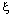) is
(2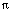)-1/2 exp(i k).
10. Consider the two-dimensional heat equation ut =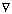2 u, on the half-space where y > x.
(a) Find the fundamental solution for this PDE with zero Dirichlet boundary conditions, i.e., u(t;x,x) = 0. (b) Solve the initial-boundary value problem with u(0;x,y) = 2.